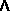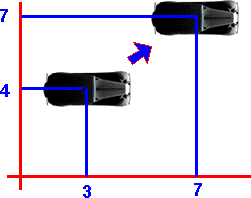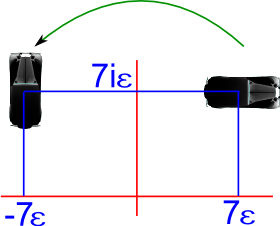# Maths - 2D Transforms using Multivectors

On this page we derived the following table summarises general transforms in any number of dimensions, here we will look at the specifics of this in two dimensions,

derivation transform
no change P/P=1 (Va * P) / P
parallel component go here (Va • P) / P
perpendicular component go here (VaP) / P
reflection go here -(P * Va) / P
rotation go here (M * Va) / M
scaling   Va * s
translation   ?
intersections an unions   ?

Notes:

• Here I have shown the inverse as "/P" because the order is not ambigous but if there is any doubt it is better to use P-1.
• Va is the input vector
• P is the transform vector
• M is the transform multivector (even grades)
• These transforms are all symmetrical around the origin, we are not yet introducing translations.

## Reflections

Lets start with very specific cases, such as how the basis vectors are transformed, this will explain the basic principles we can then go on to the more general case.

then:

• The basis vectors are e1, e2
• e1e1 = e2e2 = +1 (assuming space vectors square to +1)
• e1 e2 = -e2 e1 (vectors anti-commute)

So, first, how are the basis vectors transformed individually?

Let n = e1 = normal to mirror (so mirror is along e2 plane)

So the basis vectors transformed by the mirror as follows:
e1' = -e1 e1 e1 = -e1
e2' = -e1 e2 e1 = +e2 e1 e1= +e2

So as we would expect, components of vectors parallel to the mirror are unchanged, components of vectors perpendicular to the mirror are inverted.

Now for the more general case, the normal to the first mirror is:
(a e1 + b e2)

so e1 transforms to:
e1 -> -(a e1 + b e2) e1 (a e1 + b e2)
= -(a + b e2 e1) (a e1 + b e2)
= -a² e1 - a b e2 - a b e2 - b² e2e1e2
= -2 a b e2 - a² e1 - b² e2e1e2
= -2 a b e2 + (b² - a²) e1

so e2 transforms to:
e2 -> -(a e1 + b e2) e2 (a e1 + b e2)
= -(a e1e2 + b) (a e1 + b e2)
= a² e2 - a b e1 - a b e1 - b² e2
= -2 a b e1 + a² e1 - b² e2
= -2 a b e1 + (a² - b²) e2

which is the same result as the matrix:

 -Px2 + Py* Py - 2 * Px * Py - 2 * Py * Px -Py2 + Px*Px

#### Reflections in 2D non-Eulidean space

So now lets look at how reflections act in non-euclidean spaces, in this case we must use the -P*Va*P-1 form, so if for instance e1² = e2² = -1 then we get:

e1' = -e1 e1 e1-1 = e1-1 = e1 /(e1*e1)=-e1

type P
direction
Va
point being reflected
Result
e1²=+1
e2²=+1
e1 e1 -e1
e1 e2 e2
a e1 + b e2 e1 -2 a b e2 + (b² - a²) e1
a e1 + b e2 e2 -2 a b e1 + (a² - b²) e2
e1²=-1
e2²=-1
e1 e1 -e1
e1 e2 e2
a e1 + b e2 e1 -2 a b e2 + (b² - a²) e1
a e1 + b e2 e2 -2 a b e1 + (a² - b²) e2
e1²=+1
e2²=-1
e1 e1 -e1
e1 e2 e2
a e1 + b e2 e1 -2 a b e2 + (b² - a²) e1
a e1 + b e2 e2 -2 a b e1 + (a² - b²) e2

So to summarise the results:

• The reflections reverse one basis vector.
• The results are independant of whether the basis vectors square to +ve or -ve. (provided we use the -P*Va*P-1 form)
• The results work for a linear combination of basis vectors to represent a reflection in any line through the origin.

## Representing 2D rotations

As we derived here the mapping to rotate the point 'p' is:

p' = M * p * M-1

Where:

M = is a multivector representing the product of two vectors and so contains only the scalar and bivector terms (even subalgebra) it is equivalent to (isomorphic to) complex number algebra. we can show this as follows:

(a e1 + b e2)(c e1 + d e2) = (ac+bd) + (ad-bc) e12

Since the scalar part square to +ve and e12 squares to -ve this is the same as a complex number.

As we did with reflection we can see how are the basis vectors transformed individually and then the general case.

So, assuming e1e1 = e2e2 = +1, the basis vectors transformed by the scalar 's' and bivector e12 individually as follows:

rotation in pure scalar:
e1' = s e1 s-1 = e1
e2' = s e2 s-1 = e2

which is a null rotation.

rotation in pure bivector:
e1' = e12 e1 e12-1 = -e21e1e21 = -e1
e2' = e12 e2 e12-1 = e1e2e1 = -e2

So we can see that rotation reverses an even number of basis vectors and therefore does not alter left or right-handedness as we would expect.

For the more general case, the normal to the first mirror is:
(a + b e12)

so e1 transforms to:
e1 -> (a + b e12) e1 (a + b e12)-1
= (a e1 - b e2) (a - b e12)/(a + b e12)(a - b e12)
= a² e1 - a b e2 - a b e2 - b² e1
= (a² - b²) e1 - 2ab e2

so e2 transforms to:
e2 -> (a + b e12) e2 (a + b e12)-1
= (a e2 + b e1) (a - b e12)
= a² e2 - a b e1 - a b e1 - b² e2
= - 2ab e1 + (a² - b²) e2

We will start with pure rotations using a conventional algebra where both the vectors square to +ve. This is just a 2 dimensional version of the more general rotation formula on this page.

We will then go on use 2D GA to represent transforms to represent isometries, to do this we need to use an algebra where one vector squares to -ve and the other vector squares to zero. This is equivalent to (isomorphic to) Dual Complex Numbers.

On this page we saw that we can use the 'sandwich' product to execute a transform as follows:

Pout = M Pin M-1

where:

• M = a multivector which defines the transform
• Pin = an object to be transformed, for example, a point.
• M-1 = the multiplicative inverse
• Pout = the result of the transform

A 2D rotation is represented by the product of two 2D vectors (a e1 + b e2)(c e1 + d e2) which gives one scalar term and one bivector term (e12), this is equivalent (isomorphic to) complex numbers. Note the odd grades (vectors in this case) cancel out because the dimensions anticommute.

In 2D we can greatly simplify this because the multiplicative inverse is equivalent to the reverse (described on this page).

In other words M-1 = M† ,so ,

Pout = M Pin M†

where:

• M† = the reversal of the transform, that is we reverse the order of basis vectors in each term separately.

In this case M is a linear combination of the scalar and bivector terms:

M = a + b e12

therefore:

M†= a - b e12

which gives to formula for the transform:

Pout = (a + b e12) Pin (a - b e12)

So in terms of the rotation angle θ we have:

M = cos(θ/2) + sin(θ/2) e12

Let us see what effect this has on a vector, say Pin= e1 this gives:

Pout = (a + b e12) e1 (a - b e12)

which simplifies to:

Pout = (a² - b²)e1 - 2*a b e2

in terms of θ this is:

(cos²(θ/2) - sin²(θ/2))e1 - 2*cos(θ/2)*sin(θ/2) e2

Which can be simplified using the half angle formula here to give:

cos(θ) e1 - sin(θ) e2

Which is what we would expect, there are simpler ways to do this but it is good to confirm that this method works.

## Representing 2D transforms

To represent isometry transforms in 2D we only need one scalar value to represent rotation and we need 2 scalar values (a 2D vector) to represent translation. A multivector based on 2D vectors therefore has enough values to represent a 2D isometries, for instance e12 might hold the rotation value and (e1 ,e2 ) hold the translation value, but does the algebra work out?

I can't find a way to represent rotation and translation (2D isometries) using multivectors based on two dimensions that square to positive numbers G2,0,0 (see my attempt here). However we can represent 2D rotation using G+2,0,0 (complex numbers) and 2D isometries using G 0,1,1 (dual complex numbers) as explained below:

## 2D Rotation Using G+2,0,0 (Complex Numbers)

We can represent 2D rotations using complex numbers as explained on this page.

## 2D Rotation and Translation Using G 0,1,1 (Dual Complex Numbers)

This can be used to represent the group of spatial displacements in two dimensions SE(2) which can be used to represent a combination of 2D rotation and displacement. If you would prefer to do this using Dual Complex Number Notation see this page.

The advantage of this is that we can represent a combined rotation with displacement operation with one multiplication operation.

• The e and e1 terms together can represent rotation (as a normalised complex number).
• The e2 and e12 terms together can represent displacement.

In order to investigate the practicality of this we can try to use it in the car racing game (which is developed on these pages). This is used in the following examples:

### Example - Pure Displacement (no rotation)

If the rotation is 0 degrees, this is represented by (e + 0 e1), in other words 1, as explained on this page.

So if we are initially at point (x=3, y=4) this will be represented by the Dual Complex Number:

(e + 3e2 + 4 e12)

We now want to displace this by (x=4, y=3) this will be represented by the Dual Complex Number:

(e + 4e2 + 3 e12)So to combine these, to give the resulting position, we multiply together their representations:

(e + 3e2 + 4 e12)*(e + 4e2 + 3 e12)

multiplying out the terms using the above multiplication table we get:

= e + 4e2 + 3 e12 + 3e2 + 4 e12

= e + 7e2 + 7 e12

### Example - Pure Rotation (no displacement)

If the displacement is zero then e2 = 0 and e12 =0 and the rotation is represented by the normalised complex number as explained on this page.

### Example - Combined Displacement and Rotation

Starting from the previous position:

(e + 7e2 + 7 e12)

and applying a rotation of 90 represented by:

(0 e + e1)We combine them by multiplying as before:

(0 e + e1) * (e + 7e2 + 7 e12)

multiplying out the terms using the above multiplication table we get:

(e1 - 7 e2 + 7 e12)

So we can see that the object is rotated about the origin.

Where I can, I have put links to Amazon for books that are relevant to the subject, click on the appropriate country flag to get more details of the book or to buy it from them.Geometric Algebra for Physicists - This is intended for physicists so it soon gets onto relativity, spacetime, electrodynamcs, quantum theory, etc. However the introduction to Geometric Algebra and classical mechanics is useful.